# Unsupervised Learning NEt WORKS PCA NETWORK PCA is

• Slides: 23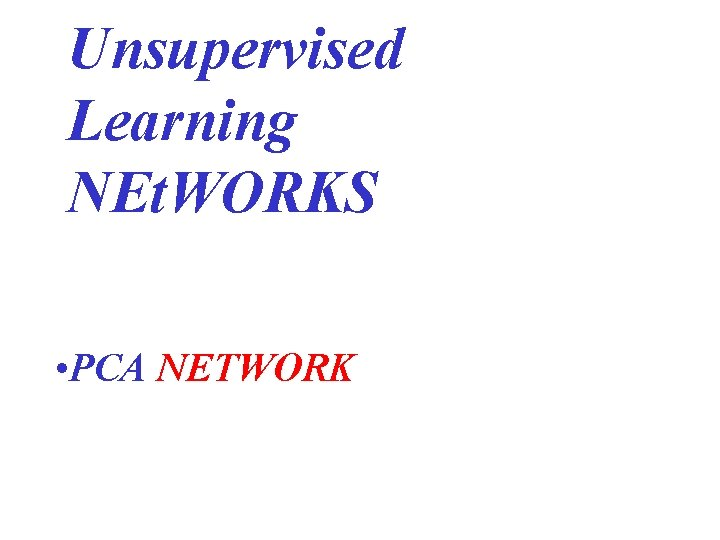Unsupervised Learning NEt. WORKS • PCA NETWORKPCA is a Representation Network useful for signal, image, video processingPCA NEt. WORKS In order to analyze multi-dimensional input vectors, a representation with maximum information is the principal component analysis (PCA). PCA • per component: extract most significant features, • inter-component: avoid duplication or redundancy between the neurons.An estimate of the autocorrelation matrix by taking the time average over the sample vectors: Rx Řx = (1/M ) Σt x(t)xt(t) Rx = t UΛUthe optimal matrix W is formed by the first m singular vectors of Rx. x(t) = W a(t) the errors of the optimal estimate are [Jain 89]: • matrix-2 -norm error = λm+1 • least-mean-square error = Σin=m+1 λi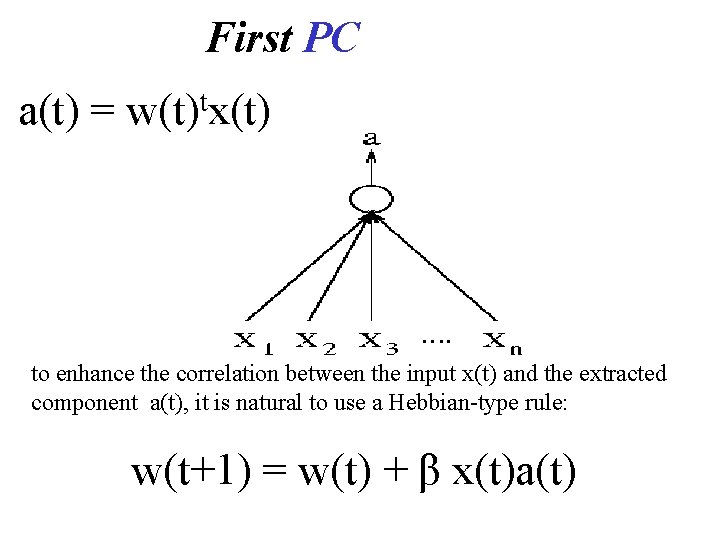First PC a(t) = t w(t) x(t) to enhance the correlation between the input x(t) and the extracted component a(t), it is natural to use a Hebbian-type rule: w(t+1) = w(t) + β x(t)a(t)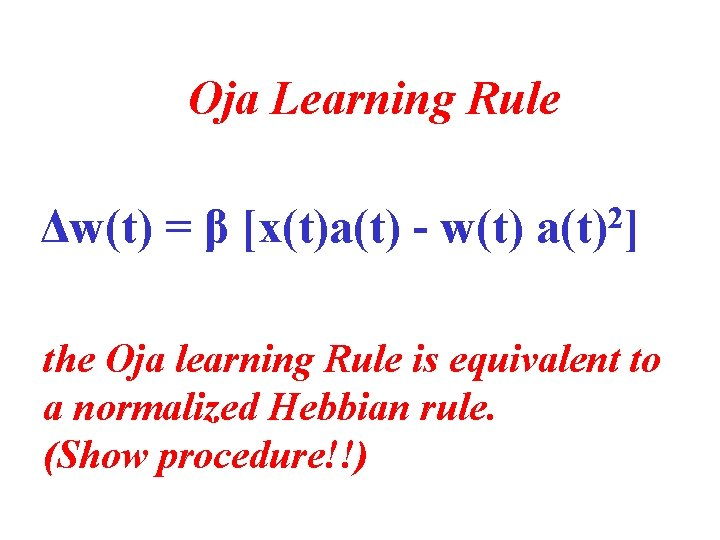Oja Learning Rule Δw(t) = β [x(t)a(t) - w(t) 2 a(t) ] the Oja learning Rule is equivalent to a normalized Hebbian rule. (Show procedure!!)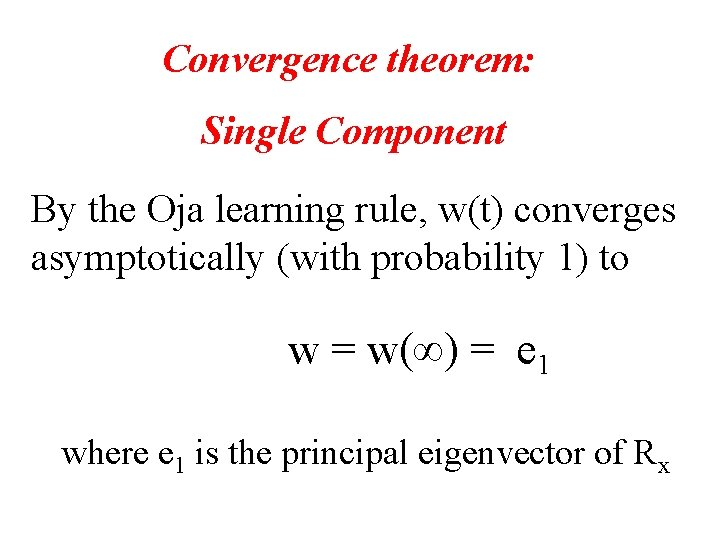Convergence theorem: Single Component By the Oja learning rule, w(t) converges asymptotically (with probability 1) to w = w(∞) = e 1 where e 1 is the principal eigenvector of Rx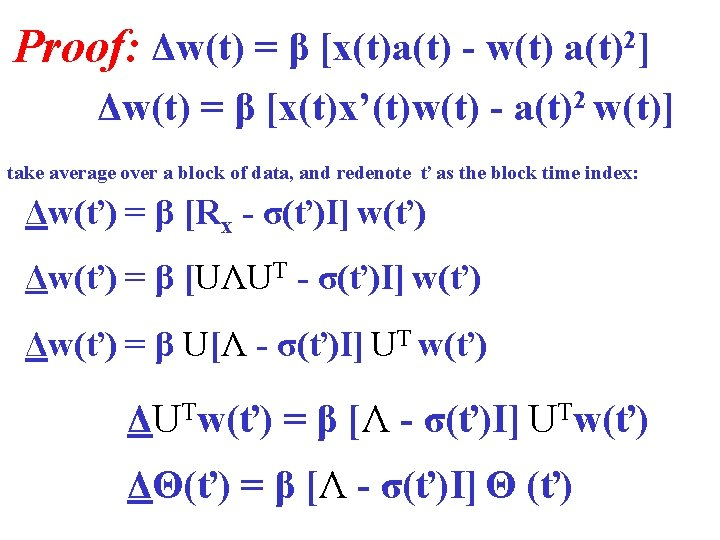Proof: Δw(t) = β [x(t)a(t) - w(t) a(t)2] Δw(t) = β [x(t)x’(t)w(t) - a(t)2 w(t)] take average over a block of data, and redenote ť as the block time index: Δw(ť) = β [Rx - σ(ť)I] w(ť) Δw(ť) = β [UΛUT - σ(ť)I] w(ť) Δw(ť) = β U[Λ - σ(ť)I] UT w(ť) ΔUTw(ť) = β [Λ - σ(ť)I] UTw(ť) ΔΘ(ť) = β [Λ - σ(ť)I] Θ (ť)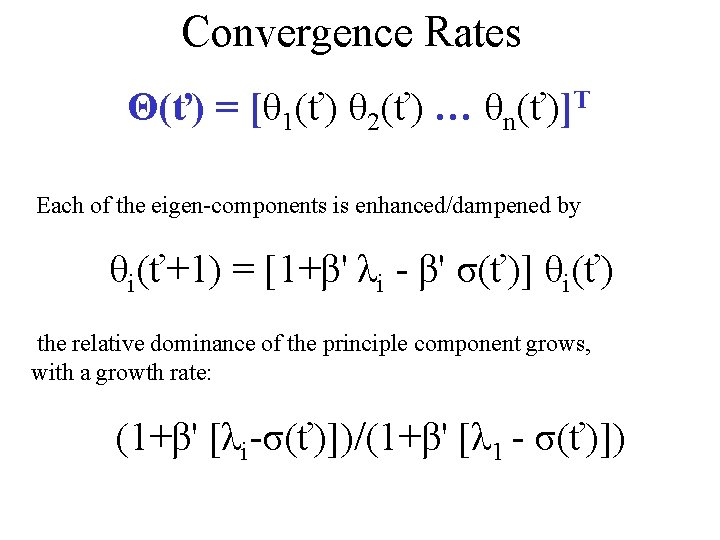Convergence Rates Θ(ť) = [θ 1(ť) θ 2(ť) … θn(ť)]T Each of the eigen-components is enhanced/dampened by θi(ť+1) = [1+β' λi - β' σ(ť)] θi(ť) the relative dominance of the principle component grows, with a growth rate: (1+β' [λi-σ(ť)])/(1+β' [λ 1 - σ(ť)])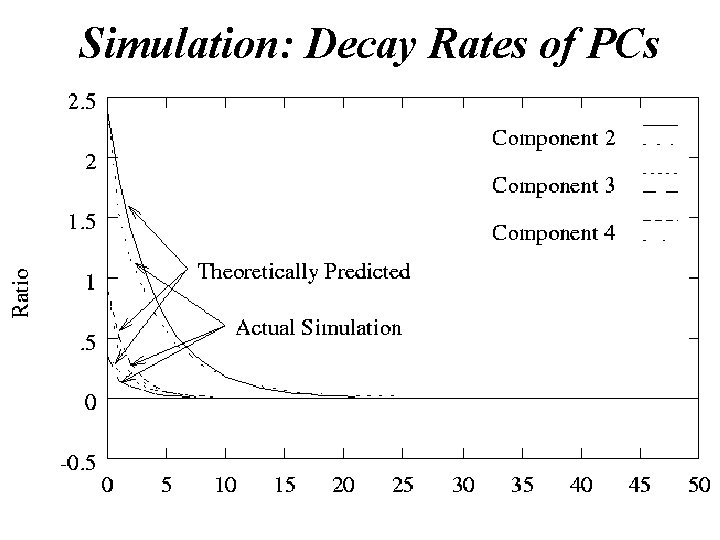Simulation: Decay Rates of PCs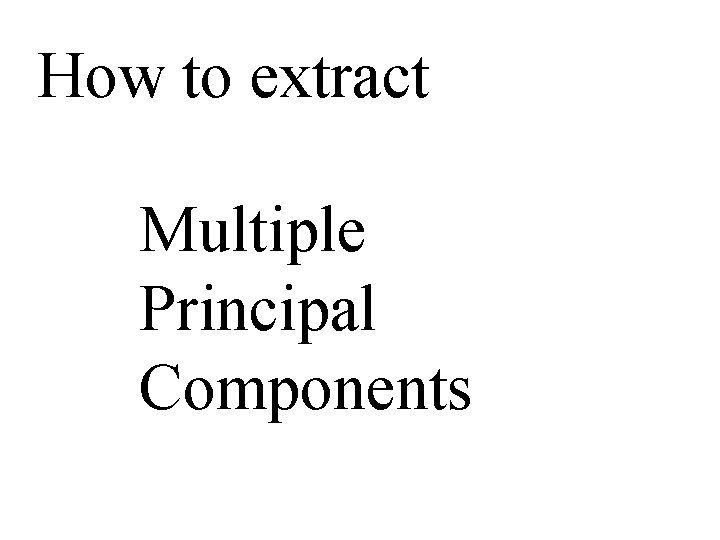How to extract Multiple Principal Components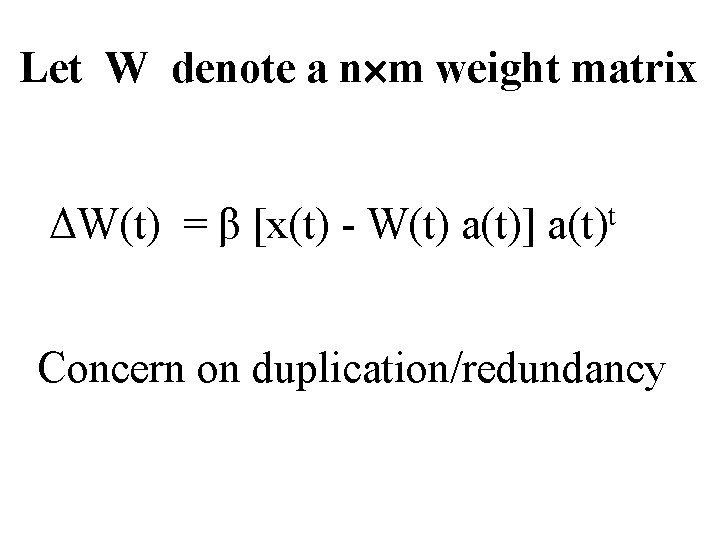Let W denote a n m weight matrix ΔW(t) = β [x(t) - W(t) a(t)] a(t)t Concern on duplication/redundancy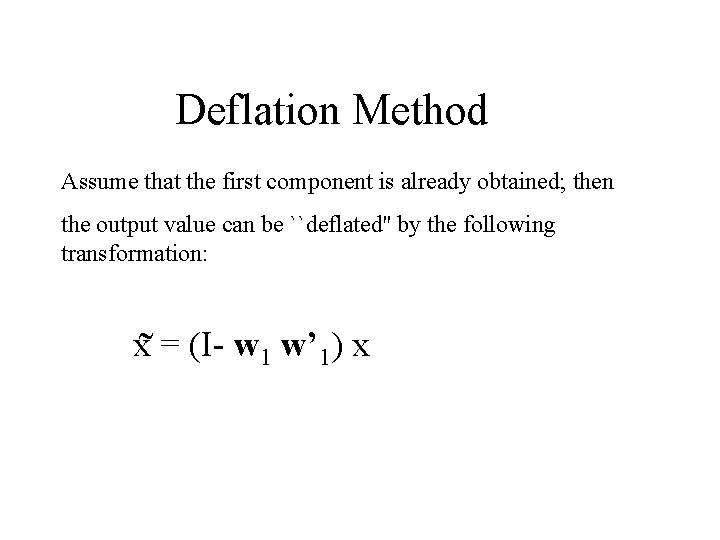Deflation Method Assume that the first component is already obtained; then the output value can be ``deflated'' by the following transformation: x˜ = (I- w 1 w’ 1) x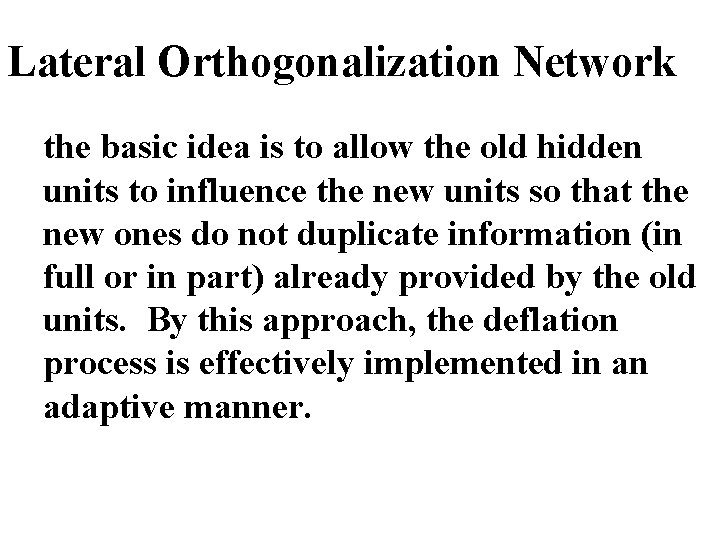Lateral Orthogonalization Network the basic idea is to allow the old hidden units to influence the new units so that the new ones do not duplicate information (in full or in part) already provided by the old units. By this approach, the deflation process is effectively implemented in an adaptive manner.APEX Network (multiple PCs)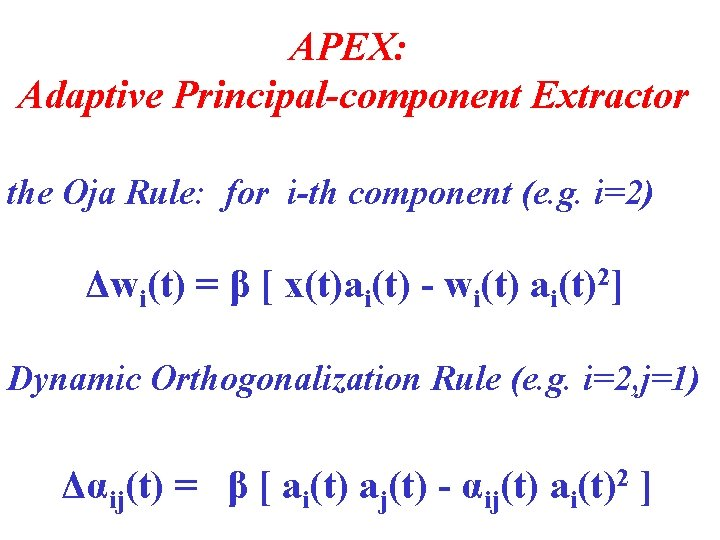APEX: Adaptive Principal-component Extractor the Oja Rule: for i-th component (e. g. i=2) Δwi(t) = β [ x(t)ai(t) - wi(t) ai(t)2] Dynamic Orthogonalization Rule (e. g. i=2, j=1) Δαij(t) = β [ ai(t) aj(t) - αij(t) ai(t)2 ]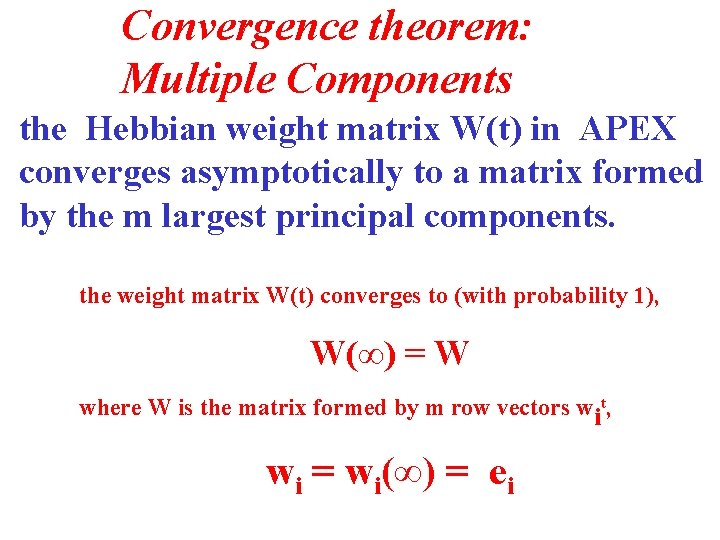Convergence theorem: Multiple Components the Hebbian weight matrix W(t) in APEX converges asymptotically to a matrix formed by the m largest principal components. the weight matrix W(t) converges to (with probability 1), W(∞) = W where W is the matrix formed by m row vectors wit, wi = wi(∞) = ei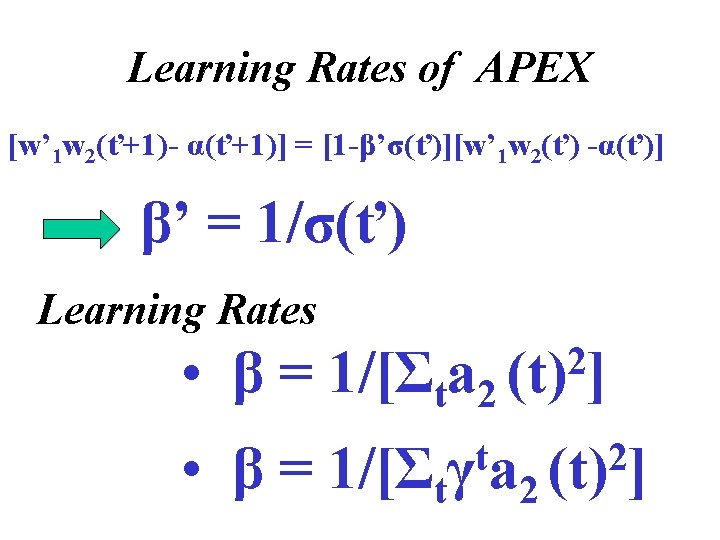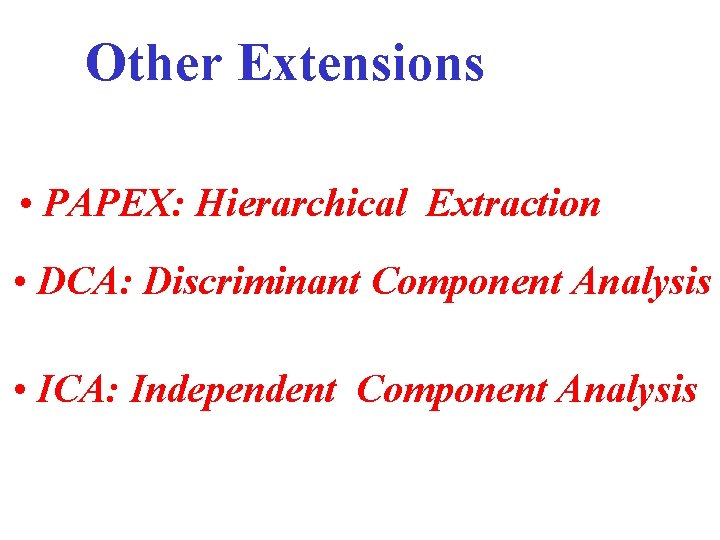Other Extensions • PAPEX: Hierarchical Extraction • DCA: Discriminant Component Analysis • ICA: Independent Component Analysis